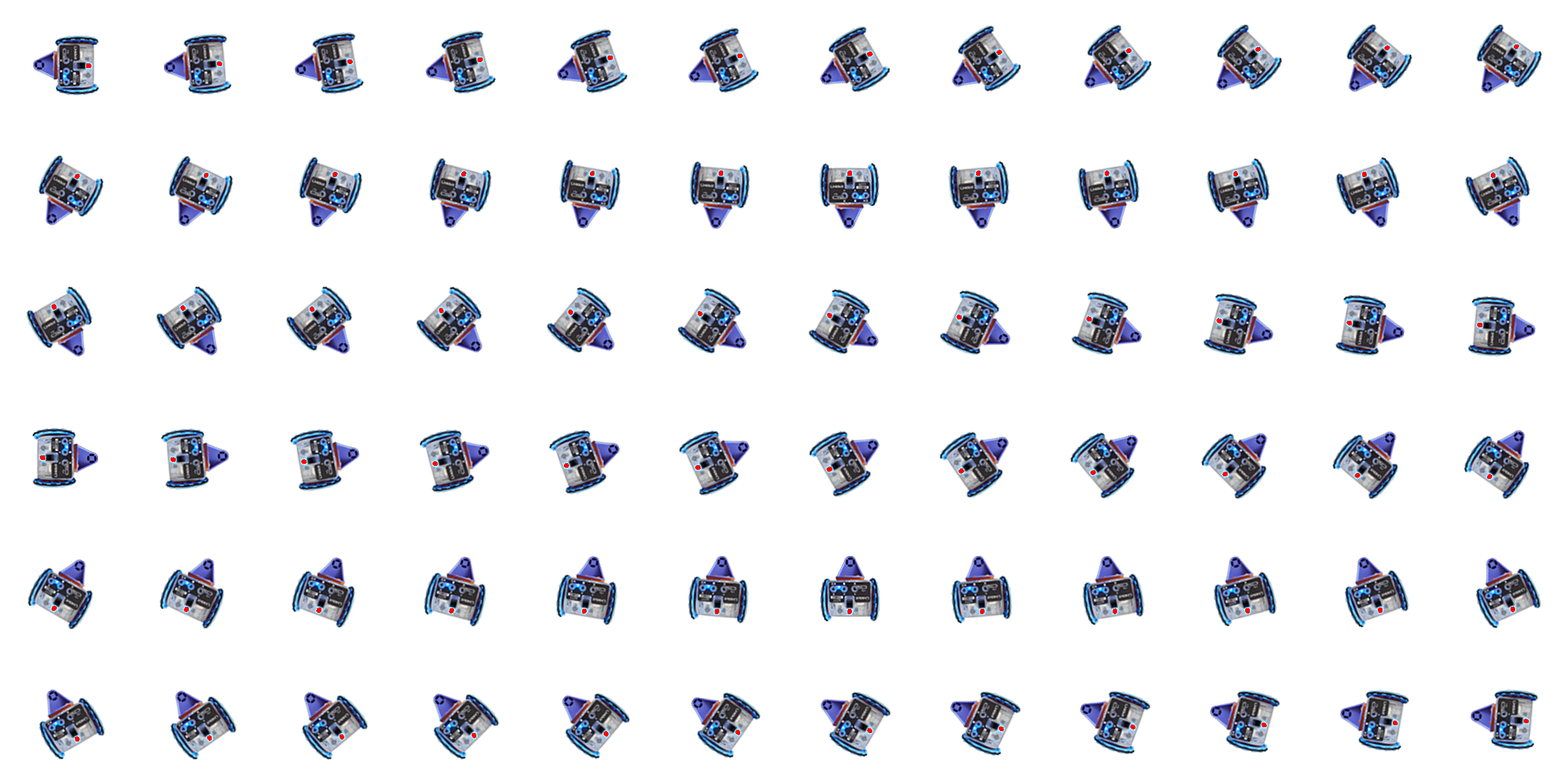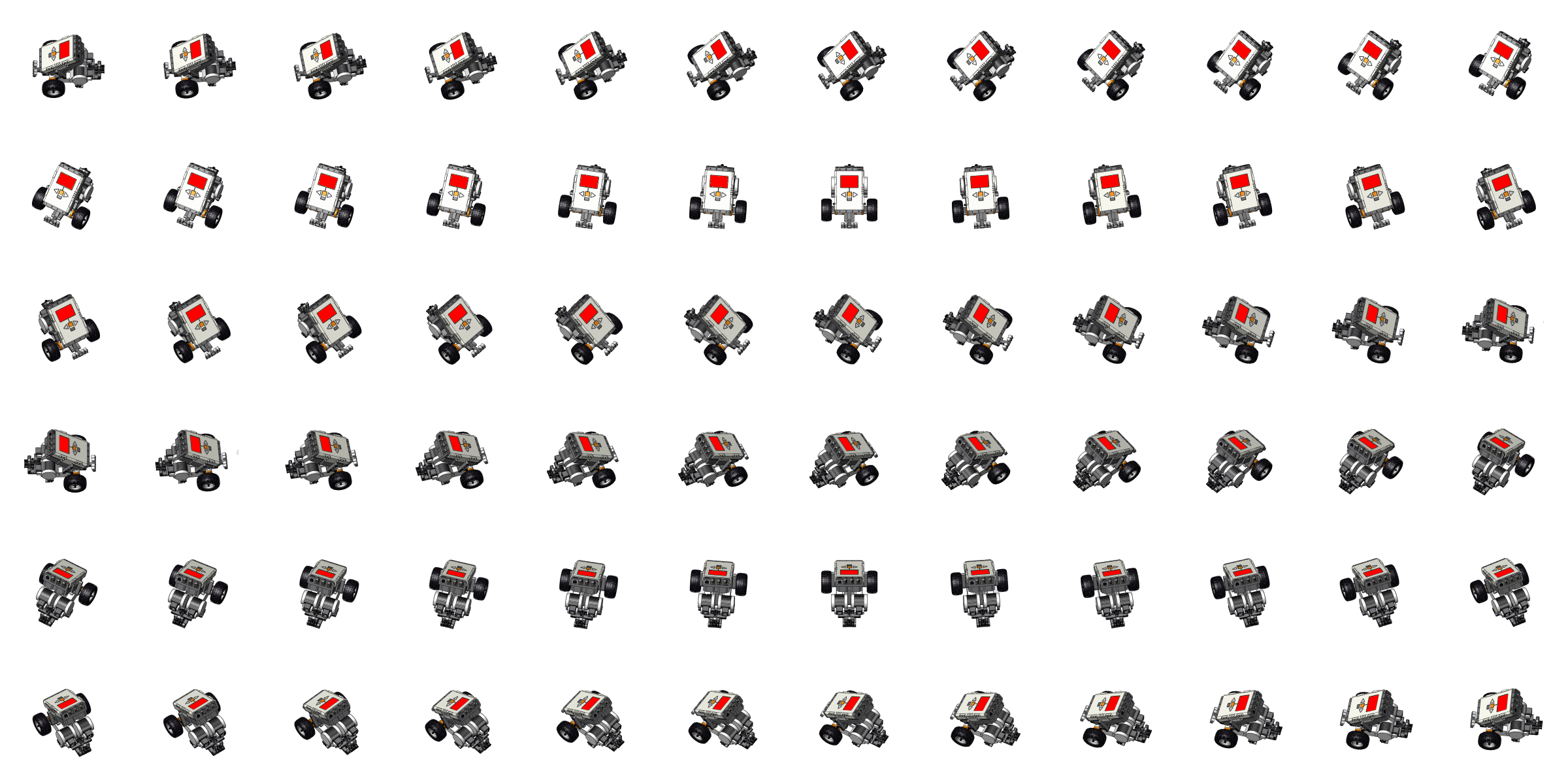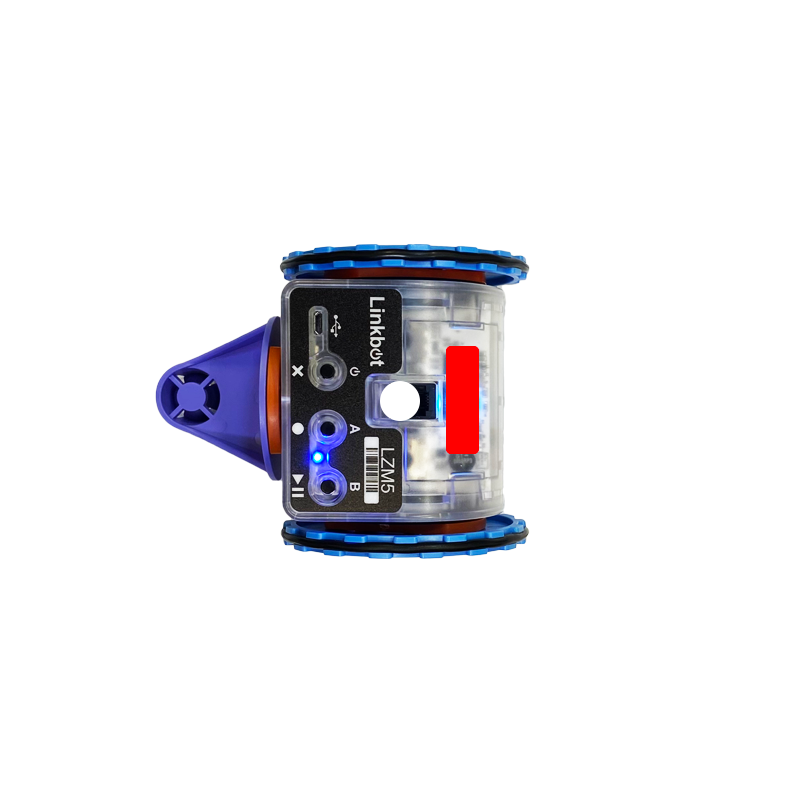Learning Math with Coding and RoboticsGrid Size: S M L Simple View: MinView:Starting Position (x, y): ( , ) in Starting Angle: degrees Current Position (x, y): (0, 0) in Current Angle: 90 degrees Wheel Radius: 1.75 in1.625 in2.0 in
Width pxStarting Position (x, y): ( , ) in Starting Angle: degrees Current Position (x, y): (0, 12) in Current Angle: 0 degrees Wheel Radius: 1.75 in1.625 in2.0 in
Width pxStarting Position (x, y): ( , ) in Starting Angle: degrees Current Position (x, y): (0, 18) in Current Angle: 0 degrees Wheel Radius: 1.75 in1.625 in2.0 in
Width px